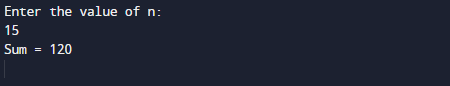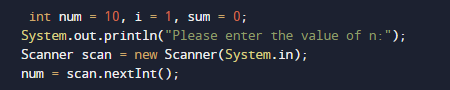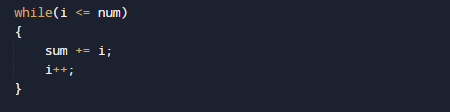# Java Program to Find sum of N Natural numbers

In this tutorial you will learn about the Java Program to Find sum of N Natural numbers and its application with practical example.

In this tutorial, we will learn to create a Java Program to Find sum of N Natural numbers   using Java programming.

## Prerequisites

Before starting with this tutorial we assume that you are best aware of the following Java programming topics:

• Java Operators.
• Basic Input and Output
• Class and Object.
• Basic Java programming.
• Loop in Java
• While loop For loops.

## What Is Natural  Number?

Mathematically ,Natural numbers are those numbers used for counting and ordering,Simply all the counting numbers  {1, 2,3.4…} are commonly called Natural numbers.Natural numbers  include all the positive integers from 1 to infinity.

## Java Program to Calculate the Sum of Natural Numbers

Firstly we declare required header file and variable and also initiates required values in variable. Next we take value from user at run time and then after we will find the sum of all N natural number of given number.

## OutputIn our program we iterate from 1 to the given (15) and adds all numbers to the variable Sum
sum=sum+i. and after calculating value we will print sum or all natural number.In the above program, we have first declared and initialized a set variables required in the program.

• number=to take value from user.
• i =For iteration.
• sum = for counting sum of numbers.

In the above program, we have first declared and initialized a set variables required in the program. In our  program first we will take a value from user.After that we will start loop from 1 to given number.Within the while loop, we add all values in variable Sum .After calculating values  of all natural number between the given numbers natural number.and finally we print sum of the all “N” Natural number between 1 to given number.In this tutorial we have learn about the Java Program to Find sum of N Natural numbers and its application with practical example. I hope you will like this tutorial.